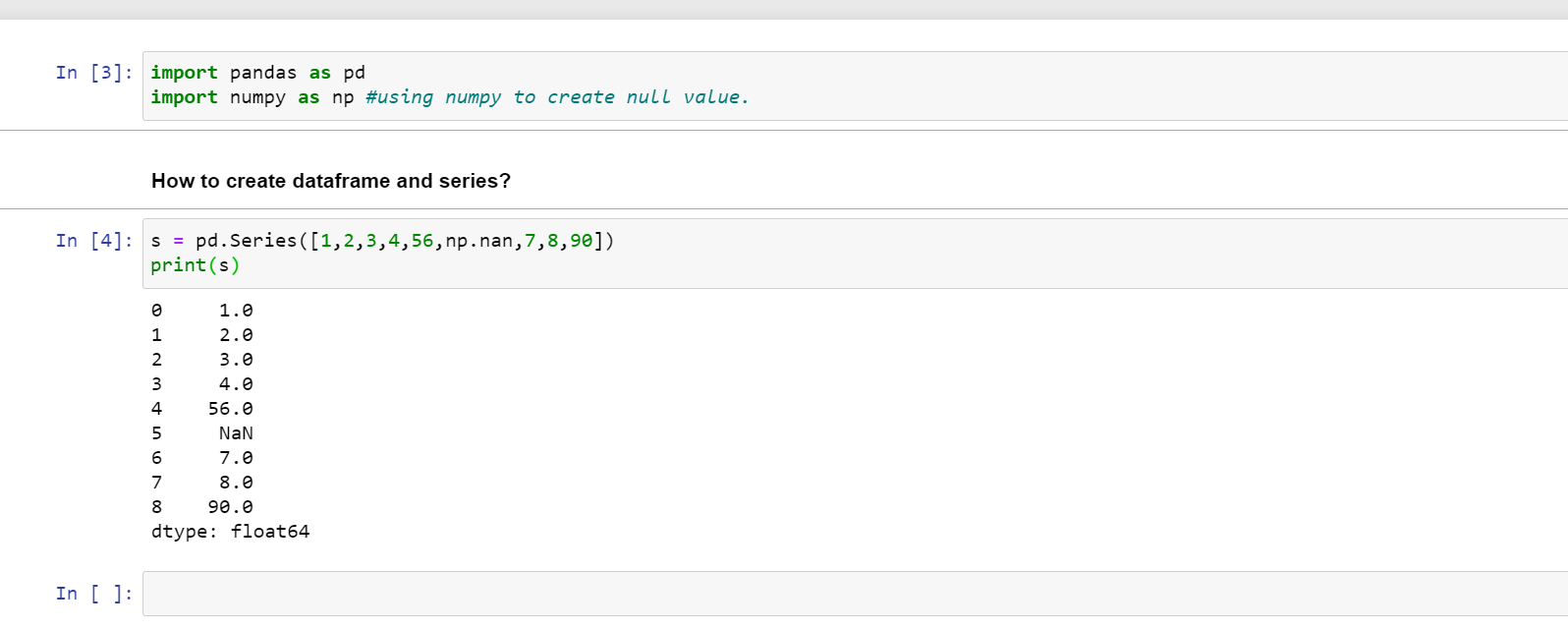1578665580

# Python Pandas Tutorial: DataFrame and Series Basics

In this video, we will be learning about the Pandas DataFrame and Series objects.

In this Python Programming video, we will be learning about the DataFrame and Series objects. These are the backbone of Pandas and are fundamental to the library. DataFrames can be thought of as rows and columns, while a Series can be thought of as just a single column of rows. We’ll also learn the basic navigation of these datatypes by learning how to select specific rows and columns. Let’s get started…

The code for this video can be found at: http://bit.ly/Pandas-02

#python #pandas

## Buddha Community1619518440

## top 30 Python Tips and Tricks for Beginners

Welcome to my Blog , In this article, you are going to learn the top 10 python tips and tricks.

### 8) Check The Memory Usage Of An Object.

#python #python hacks tricks #python learning tips #python programming tricks #python tips #python tips and tricks #python tips and tricks advanced #python tips and tricks for beginners #python tips tricks and techniques #python tutorial #tips and tricks in python #tips to learn python #top 30 python tips and tricks for beginners

1586702221

## Python Pandas Objects - Pandas Series and Pandas Dataframe

In this post, we will learn about pandas’ data structures/objects. Pandas provide two type of data structures:-

### Pandas Series

Pandas Series is a one dimensional indexed data, which can hold datatypes like integer, string, boolean, float, python object etc. A Pandas Series can hold only one data type at a time. The axis label of the data is called the index of the series. The labels need not to be unique but must be a hashable type. The index of the series can be integer, string and even time-series data. In general, Pandas Series is nothing but a column of an excel sheet with row index being the index of the series.

### Pandas Dataframe

Pandas dataframe is a primary data structure of pandas. Pandas dataframe is a two-dimensional size mutable array with both flexible row indices and flexible column names. In general, it is just like an excel sheet or SQL table. It can also be seen as a python’s dict-like container for series objects.

#python #python-pandas #pandas-dataframe #pandas-series #pandas-tutorial1625827020

## Understanding Pandas Series - Python Pandas Tutorial #2

Series is a crucial pandas data structure that you need to master in order to get information of your dataFrame. We will cover from the very basic to more advanced concepts in 15 minutes. We will go through different ways to create a series, how to access and set values, how to slice using index and label index, how to perform math operations and advanced selection using boolean filter.

0:00 - Intro
0:09 - Pandas data structures
0:25 - What is a pandas Series
1:23 - How to create series
4:11 - How to access and set elements to a pandas Series
6:26 - How to slice to a pandas Series
8:34 - Boolean filter selection
12:02 - Arithmetic operations on Series
14:20 - Notebooks on Github

► DATA SCIENCE COURSES
• Learn how to use Jupyter Notebooks ➭ https://www.youtube.com/watch?v=gGYaFfAvYtg

► PYTHON PROGRAMMING COURSES
• Beginner Python Tutorials ➭ https://www.youtube.com/watch?v=HG_E6EaKY90&list=PLJgwF35R54crXsGuSKR_MtUG2ABU_BFAq
• Intermediate Python Tutorials ➭ https://www.youtube.com/watch?v=oNwaOFZDAWo&list=PLJgwF35R54coNbQXGNJyawp-_3CC6I1B4

► SOCIAL MEDIA

#pandas #python #python pandas tutorial #pandas series1602550800

## Pandas in Python

Pandas is used for data manipulation, analysis and cleaning.

What are Data Frames and Series?

Dataframe is a two dimensional, size mutable, potentially heterogeneous tabular data.

It contains rows and columns, arithmetic operations can be applied on both rows and columns.

Series is a one dimensional label array capable of holding data of any type. It can be integer, float, string, python objects etc. Panda series is nothing but a column in an excel sheet.

### How to create dataframe and series?

s = pd.Series([1,2,3,4,56,np.nan,7,8,90])

print(s)How to create a dataframe by passing a numpy array?

1. d= pd.date_range(‘20200809’,periods=15)
2. print(d)
3. df = pd.DataFrame(np.random.randn(15,4), index= d, columns = [‘A’,’B’,’C’,’D’])
4. print(df)

#pandas-series #pandas #pandas-in-python #pandas-dataframe #python1619510796

## Lambda, Map, Filter functions in python

Welcome to my Blog, In this article, we will learn python lambda function, Map function, and filter function.

Lambda function in python: Lambda is a one line anonymous function and lambda takes any number of arguments but can only have one expression and python lambda syntax is

Syntax: x = lambda arguments : expression

Now i will show you some python lambda function examples:

#python #anonymous function python #filter function in python #lambda #lambda python 3 #map python #python filter #python filter lambda #python lambda #python lambda examples #python map